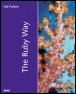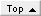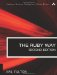# Working with TreesRuby Way By Hal Fulton Slots : 1.0 Table of Contents

I think that I shall never see

A poem as lovely as a tree….

[Alfred] Joyce Kilmer, "Trees"

A tree in computer science is a relatively intuitive concept (except that it is usually drawn with the "root" at the top and the "leaves" at the bottom). This is because we are familiar with so many kinds of hierarchical data in everyday lifefrom the family tree, to the corporate org chart, to the directory structures on our hard drives.

The terminology of trees is rich but easy to understand. Any item in a tree is a node; the first or topmost node is the root. A node may have descendants that are below it, and the immediate descendants are called children. Conversely, a node may also have a parent (only one) and ancestors. A node with no child nodes is called a leaf. A subtree consists of a node and all its descendants. To travel through a tree (for example, to print it out) is called traversing the tree.

We will look mostly at binary trees, although in practice a node can have any number of children. You will see how to create a tree, populate it, and traverse it. Also, we will look at a few real-life tasks that use trees.

We should mention here that in many languages, such as C and Pascal, trees are implemented using true address pointers. However, in Ruby (as in Java, for instance), we don't use pointers; object references work just as well or even better.

#### Implementing a Binary Tree

There is more than one way to implement a binary tree in Ruby. For example, we could use an array to store the values. Here, we use a more traditional approach, coding much as we would in C, except that pointers are replaced with object references.

What is required in order to describe a binary tree? Well, each node needs an attribute of some kind for storing a piece of data. Each node also needs a pair of attributes for referring to the left and right subtrees under that node.

We also need a way to insert into the tree and a way of getting information out of the tree. A pair of methods will serve these purposes.

The first tree we'll look at will implement these methods in a slightly unorthodox way. We will expand on the Tree class in later examples.

A tree is, in a sense, defined by its insertion algorithm and by how it is traversed. In this first example, shown in Listing 3.16, we define an insert method that inserts in a breadth-first fashion (that is, top to bottom and left to right). This guarantees that the tree grows in depth relatively slowly and is always balanced. Corresponding to the insert method, the traverse iterator will iterate over the tree in the same breadth-first order.

##### Listing 3.16 Breadth-First Insertion and Traversal in a Tree
` class Tree attr_accessor :left attr_accessor :right attr_accessor :data def initialize(x=nil)   @left = nil   @right = nil   @data = x end def insert(x)   list = []   if @data == nil     @data = x   elsif @left == nil     @left = Tree.new(x)   elsif @right == nil     @right = Tree.new(x)   else     list << @left     list << @right     loop do       node = list.shift       if node.left == nil         node.insert(x)         break       else         list << node.left       end       if node.right == nil         node.insert(x)         break       else         list << node.right       end     end   end end def traverse()   list = []   yield @data   list << @left if @left != nil   list << @right if @right != nil   loop do     break if list.empty?     node = list.shift     yield node.data     list << node.left if node.left != nil     list << node.right if node.right != nil   end end end items = [1, 2, 3, 4, 5, 6, 7] tree = Tree.new items.each { |x| tree.insert(x)} tree.traverse { |x| print "#{ x}  "} print "\n" # Prints "1 2 3 4 5 6 7 " `

This kind of tree, as defined by its insertion and traversal algorithms, is not especially interesting. However, it does serve as an introduction and something on which we can build.

#### Sorting Using a Binary Tree

For random data, using a binary tree is a good way to sort. (Although in the case of already-sorted data, it degenerates into a simple linked list.) The reason, of course, is that with each comparison, we are eliminating half the remaining alternatives as to where we should place a new node.

Although it might be fairly rare to sort using a binary tree nowadays, it can't hurt to know how. The code in Listing 3.17 builds on the previous example.

##### Listing 3.17 Sorting with a Binary Tree
` class Tree   # Assumes definitions from   # previous example...   def insert(x)     if @data == nil       @data = x     elsif x <= @data       if @left == nil         @left = Tree.new x       else         @left.insert x       end     else       if @right == nil         @right = Tree.new x           else             @right.insert x           end         end       end       def inorder()         @left.inorder { |y| yield y}  if @left != nil         yield @data         @right.inorder { |y| yield y}  if @right != nil       end       def preorder()         yield @data         @left.preorder { |y| yield y}  if @left != nil         @right.preorder { |y| yield y}  if @right != nil       end       def postorder()         @left.postorder { |y| yield y}  if @left != nil         @right.postorder { |y| yield y}  if @right != nil         yield @data       end end items = [50, 20, 80, 10, 30, 70, 90, 5, 14,          28, 41, 66, 75, 88, 96] tree = Tree.new items.each { |x| tree.insert(x)} tree.inorder { |x| print x, " "} print "\n" tree.preorder { |x| print x, " "} print "\n" tree.postorder { |x| print x, " "} print "\n" `

#### Using a Binary Tree As a Lookup Table

Suppose we have a tree already sorted. Traditionally, this has made for a good lookup table; for example, a balanced tree of a million items would take no more than 20 comparisons (the depth of the tree or log base 2 of the number of nodes) to find a specific node. For this to be useful, we assume that the data for each node is not just a single value but has a key value and other information associated with it.

In most if not all situations, a hash or even an external database table will be preferable. However, we present this code to you anyhow (see Listing 3.18).

##### Listing 3.18 Searching a Binary Tree
` class Tree   # Assumes definitions   # from previous example...   def search(x)     if self.data == x       return self     else       ltree = left != nil ? left.search(x) : nil       return ltree if ltree != nil       rtree = right != nil ? right.search(x) : nil       return rtree if rtree != nil     end     nil   end end keys = [50, 20, 80, 10, 30, 70, 90, 5, 14,         28, 41, 66, 75, 88, 96] tree = Tree.new keys.each { |x| tree.insert(x)} s1 = tree.search(75)   # Returns a reference to the node                        # containing 75... s2 = tree.search(100)  # Returns nil (not found) `

#### Converting a Tree to a String or Array

The same old tricks that allow us to traverse a tree will allow us to convert it to a string or array if we wish, as shown in Listing 3.19. Here, we assume an inorder traversal, although any other kind could be used.

##### Listing 3.19 Converting a Tree to a String or Array
` class Tree   # Assumes definitions from   # previous example...   def to_s     "[" +     if left then left.to_s + "," else "" end +     data.inspect +     if right then "," + right.to_s else "" end + "]"   end   def to_a     temp = []     temp += left.to_a if left     temp << data     temp += right.to_a if right     temp   end end items = %w[bongo grimace monoid jewel plover nexus synergy] tree = Tree.new items.each { |x| tree.insert x} str = tree.to_s * "," # str is now "bongo,grimace,jewel,monoid,nexus,plover,synergy" arr = tree.to_a # arr is now: # ["bongo",["grimace",[["jewel"],"monoid",[["nexus"],"plover", #  ["synergy"]]]]] `

Note that the resulting array is as deeply nested as the depth of the tree from which it came. You can, of course, use flatten to produce a non-nested array.

#### Storing an Infix Expression in a Tree

Here is another little contrived problem illustrating how a binary tree might be used (see Listing 3.20). We are given a prefix arithmetic expression and want to store it in standard infix form in a tree. (This is not completely unrealistic because the Ruby interpreter itself stores expressions in a tree structure, although it is a couple of orders of magnitude greater in complexity.)

We define a "standalone" method called addnode that will add a node to a tree in the proper place. The result will be a tree in which every leaf is an operand and every non-leaf node is an operator. We also define a new Tree method called infix, which will traverse the tree in order and act as an iterator. One twist is that it adds in parentheses as it goes, because prefix form is "parenthesis free" but infix form is not. The output would look more elegant if only necessary parentheses were added, but we added them indiscriminately to simplify the code.

##### Listing 3.20 Storing an Infix Expression in a Tree
` class Tree   # Assumes definitions from   # previous example...   def infix()     if @left != nil       flag = %w[* / + -].include? @left.data       yield "(" if flag       @left.infix { |y| yield y}       yield ")" if flag     end     yield @data     if @right != nil       flag = %w[* / + -].include? @right.data       yield "(" if flag       @right.infix { |y| yield y}  if @right != nil       yield ")" if flag     end   end end def addnode(nodes)   node = nodes.shift   tree = Tree.new node   if %w[* / + -].include? node     tree.left  = addnode nodes     tree.right = addnode nodes   end   tree end prefix = %w[ * + 32 * 21 45 - 72 + 23 11 ] tree = addnode prefix str = "" tree.infix { |x| str += x} # str is now "(32+(21*45))*(72-(23+11))" `

#### Additional Notes on Trees

We'll mention a few more notes on trees here. First of all, a tree is a special case of a graph (as you will see shortly); in fact, it is a directed acyclic graph (DAG). Therefore, you can learn more about trees by researching graph algorithms in general.

There is no reason that a tree should necessarily be binary; this is a convenient simplification that frequently makes sense. However, it is conceivable to define a multiway tree in which each node is not limited to two children but may have an arbitrary number. In such a case, you would likely want to represent the child node pointers as an array of object references.

A B-tree is a specialized form of multiway tree. It is an improvement over a binary tree in that it is always balanced (that is, its depth is minimal), whereas a binary tree in a degenerate case can have a depth that is equal to the number of nodes it has. There is plenty of information on the Web and in textbooks if you need to learn about B-trees. Also, the principles we've applied to ordinary binary trees can be extended to B-trees as well.

A red-black tree is a specialized form of binary tree in which each node has a color (red or black) associated with it. In addition, each node has a pointer back to its parent (meaning that it is arguably not a tree at all because it isn't truly acyclic). A red-black tree maintains its balance through rotations of its nodes; that is, if one part of the tree starts to get too deep, the nodes can be rearranged so that depth is minimized (and in-order traversal ordering is preserved). The extra information in each node aids in performing these rotations.

Another tree that maintains its balance in spite of additions and deletions is the AVL tree. This structure is named for its discoverers, the two Russian researchers Adel'son-Vel'skii and Landis. An AVL tree is a binary tree that uses slightly more sophisticated insertion and deletion algorithms to keep the tree balanced. It performs rotation of subtrees similar to that done for red-black trees.

All these and more are potentially useful tree structures. If you need more information, search the Web or consult any book on advanced algorithms.The Ruby Way, Second Edition: Solutions and Techniques in Ruby Programming (2nd Edition)
ISBN: 0672328844
EAN: 2147483647
Year: 2000
Pages: 119
Authors: Hal Fulton

Similar book on Amazon

flylib.com © 2008-2017.
If you may any questions please contact us: flylib@qtcs.net